Practice the AP 7th Class Maths Bits with Answers Chapter 8 Congruency of Triangles on a regular basis so that you can attempt exams with utmost confidence.

## AP State Syllabus 7th Class Maths Bits 8th Lesson Congruency of Triangles with Answers

Question 1.
Name the congruency in ΔPQR ≅ ΔABC is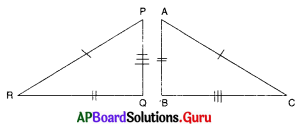A) S.A.S
B) A.S.A
C) R.H.S
D) S.S.S
D) S.S.S

Question 2.
If in ΔPEN and ΔRIM; PE = RI; ∠E = ∠I and EN = IM then
A) ΔEPN ≅ ΔRIM
B) ΔNPE ≅ ΔRIM
C) ΔPEN ≅ ΔRIM
D) ΔPEN ≅ ΔMIR
C) ΔPEN ≅ ΔRIMQuestion 3.
Which of the following pairs of figures are congruent?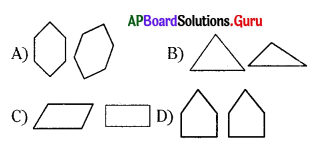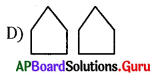Question 4.
Which of the following pair of figures are not congruent?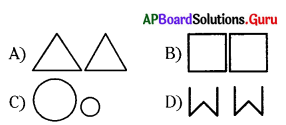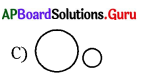Question 5.
If ΔABC ≅ ΔDEF then $$\overline{\mathbf{A C}}$$ = …………….
A) $$\overline{\mathrm{DE}}$$
B) $$\overline{\mathrm{EF}}$$
C) $$\overline{\mathrm{DF}}$$
D) None
C) $$\overline{\mathrm{DF}}$$

Question 6.
From the given figure Δ EGF ≅ ………………..?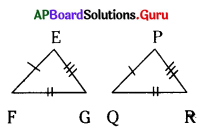A) Δ PQR
B) Δ QPR
C) Δ RPQ
D) Δ PRQ
D) Δ PRQ

Question 7.
From the above sum ΔEGF ≅ ΔPRQ then
A) ⌊E = ⌊P
B) $$\overline{\mathrm{FG}}=\overline{\mathrm{QR}}$$
C) ⌊G = ⌊R
D) All the above
D) All the above

Question 8.
If Δ CDG ≅ Δ RSW then which of the following is not correct?
A) $$\overline{\mathrm{CD}}=\overline{\mathrm{RS}}$$
B) ⌊D = ⌊R
C) $$\overline{\mathrm{DG}}=\overline{\mathrm{SW}}$$
D) ⌊G = ⌊W
B) ⌊D = ⌊R

Question 9.
From the given figure?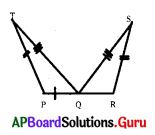A) ⌊TPQ = ⌊SQR
B) ⌊QPT = ⌊SQR
C) ⌊QPT = ⌊QSR
D) ⌊TQP = ⌊SQR
D) ⌊TQP = ⌊SQRQuestion 10.
From the given figure which of the following is true?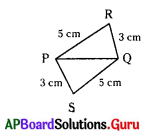A) ΔPQR ≅ ΔQPS
B) ΔPQR ≅ ΔPQS
C) ΔPSQ ≅ ΔPRQ
D) ΔPRQ ≅ ΔPQS
A) ΔPQR ≅ ΔQPS

Question 11.
From the figure then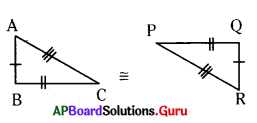A) ΔABC ≅ ΔRQP
B) ΔABC ≅ ΔPQR
C) ΔABC ≅ ΔQRP
D) ΔABC ≅ ΔRPQ
A) ΔABC ≅ ΔRQP

Question 12.
From the above sum ΔABC ≅ ΔRQP by which congruency rule?
A) S.S.A
B) A.S.A
C) S.A.S
D) S.S.S
D) S.S.S

Question 13.
ΔABC ≅ ΔDBC by which congruency rule?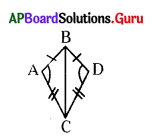A) S.A.S
B) A.S.A
C) S.S.S
D) R.H.S
A) S.A.S

Question 14.
From the given figure which corresponding parts are need to equal when ΔADC ≅ ΔABC by S.A.S rule?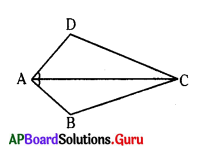A) CD = CB
B) ⌊A CB = ⌊D CA
D) ⌊D = ⌊B

Question 15.
From the given figure ΔABO ≅ ΔDCO by which congruency rule?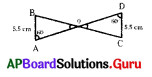A) S.A.S
B) A.S.A
C) S.S.S.
D) R.H.S
B) A.S.A

Question 16.
If ⌊M LN = ⌊F GH, ⌊N ML = ⌊G FH, ML = FG then ………………?
A) ΔLMN ≅ ΔHGF
B) ΔMNL ≅ ΔFGH
C) ΔLNM ≅ ΔHFG
D) ΔLMN ≅ ΔFGH
D) ΔLMN ≅ ΔFGHQuestion 17.
From the given figure ΔABE ≅ ΔCDB by the congruency rule of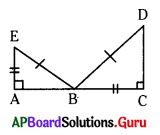A) S.A.S
B) A.S.A
C) R.H.S
D) S.S.S
C) R.H.S

Question 18.
From the given figure ΔRAT ≅ ……………….?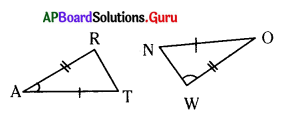A) ΔNOW
B) ΔWON
C) ΔOWN
D) ΔWNO
B) ΔWON

Question 19.
From the adjacent figure ΔTPQ ≅……………?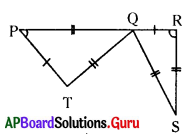A) ΔQRS
B) ΔSRQ
C) ΔQSR
D) ΔRSQ
A) ΔQRS

Question 20.
From the given figure the area of a triangle is ……………? (cms)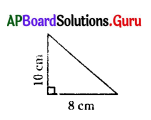A) 50
B) 40
C) 32
D) 80
B) 40

Question 21.
What is the area of a ΔABC when AB = BC = 5 cm and ⌊B = 90° (in sq cms)
A) 10
B) 6.25
C) 12.5
D) 25
C) 12.5

Question 22.
From the adjacent figure the area of ΔABC is (in sq units)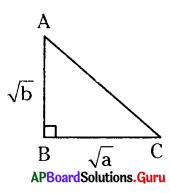A) $$\frac{1}{2}$$ab
B) $$\frac{1}{2}$$(√a)√b
C) $$\frac{1}{2}$$$$\sqrt{a b}$$
D) $$\frac{1}{2}$$(√b)√a
C) $$\frac{1}{2}$$$$\sqrt{a b}$$Question 23.
If two figures are identical and equal then they are
A) identical
B) similar
C) congruent
D) equal
C) congruent

Question 24.
Two squares are always
A) similar
B) equal
C) congruent
D) none
A) similar

Question 25.
Two circles are always
A) congruent
B) equal
C) similar
D) can’t say
C) similar

Question 26.
If 3 sides of a first triangle are correspondingly equal to 3 sides of second triangle then the two triangles are congruent under the property of …………………
A) R.H.S
B) A.S.A
C) S.A.S
D) S.S.S
D) S.S.S

Question 27.
If two sides included angle of the first triangle are correspondingly equal to two sides included angle of the second triangle then the two triangles are said to be congruent under the property of ……………..
A) R.H.S
B) A.S.A
C) S.A.S
D) S.S.S
C) S.A.S

Question 28.
Which of the following are always congruent?
A) Two circles with same radii
B) Two squares with same length of side
C) Two triangles
D) Both A & B
D) Both A & BQuestion 29.
Two line segments are congruent if they have …………….
A) same common point
B) same length
C) same angle
D) none